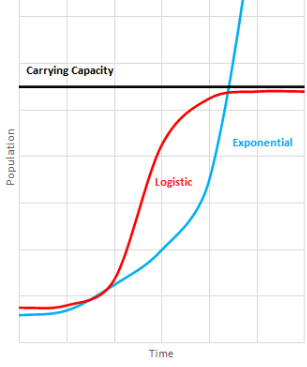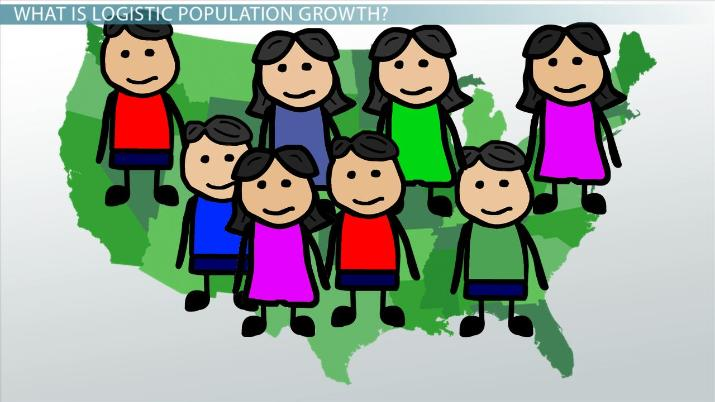# Logistic Growth Models in Population

Jack Woerner, Angela Lynn Swafford, Lesley Chapel
• Author
Jack Woerner

BA in Political Science with Emphasis on Social Studies Education at Brevard College, 6 years experience (2 years online) teaching Economics, Personal Finance, APUS Government and more. Certified Gifted/Talented Teacher.

• Instructor
Angela Lynn Swafford

Lynn has a BS and MS in biology and has taught many college biology courses.

• Expert Contributor
Lesley Chapel

Lesley has taught American and World History at the university level for the past seven years. She has a Master's degree in History.

Explore logistic growth. Understand the logistic population growth. Use the logistic growth equation and logistic growth graph to predict the population growth. Updated: 03/29/2022

Show

## What is Logistic Growth?

The logistic growth definition in population models refers to the gradual growth of the population in the beginning and then increases when the number of people grows. When does logistic growth occur? According to the logistic growth model, the population grows the most when a high number of people use the efficient number of resources available to sustain that population. In this way, logistic growth is a type of exponential growth model. The logistic growth model also shows a decrease or reduced rate of population growth as the population begins to exceed the necessary number of resources needed. This is known as exceeding the carrying capacity. Some people may not use exponential growth models because the models would not show an accurate growth rate due to the cap on resources and the exceeding of the carrying capacity.

### Logistic Growth Curve

The logistic growth curve represents the logistic population growth rate. The logistic growth curve on a line graph is S-shaped to show the slow increase, rapid population growth, and finally, the reduction in the growth.That graph shows that the exponential growth curve surpasses the carrying capacity while the logistic growth curve flattens when the population meets the carrying capacity line.

The Y-axis shows the total population increase or decreases going up and down on the graph.

The X-axis shows the advancement of time moving to the right of the graph.An error occurred trying to load this video.

Try refreshing the page, or contact customer support.

Coming up next: Neolithic Agricultural Revolution: Causes and Implications

### You're on a roll. Keep up the good work!

Replay
Your next lesson will play in 10 seconds
• 0:00 Logistic Population Growth
• 0:50 Graphing & Equation for Growth
• 2:15 Below Carrying Capacity
• 3:05 Near Carrying Capacity
• 3:35 Larger Than Carrying Capacity
• 4:05 Lesson Summary
Save Save

Want to watch this again later?

Timeline
Autoplay
Autoplay
Speed Speed

## Logistic Population Growth

Economists, mathematicians, government officials, and others would more than likely choose the logistic growth model over other population models because of the accuracy the model displays. Logistic population growth can only expand so much before the population experiences the limit of resources that can help sustain the growing population.

## Logistic Growth Equation

The logistic growth graph is created by plotting points found from the calculations involved in the logistic growth equation. The logistic growth equation components are:

• dN - Change in population
• dt - Change in time
• rN - r is the maximum per capita growth rate for a population. Per capita means per person, while the per capita growth rate is the number of births vs. death.
• K - Carrying capacity
• N - Population size
• In the logistic growth equation, the K and R values do not change over time in a population

The logistic growth equation is dN/dt=rN((K-N)/K).

A different equation can be used when an event occurs that negatively affects the population.

This equation is: f(x) = c/(1+ae^{-bx}).

Each component of this equation is:

• f(x) - population over time
• c - carrying capacity
• 1 - the starting point of the population
• a - population target or affected event
• -bx - the constant rate of growth and the time of the target event (which is usually shown as a negative because of the population decreasing

### Example

Below are examples using both logistic growth equations to find the logistic growth model.

#### f(x) = c/(1+ae^{-bx}) Example

A city of 100,000 people was infected with the coronavirus. The logistic growth rate (b) for the city is 0.8090. The city council knows that at least one person is infected and has read reports from other cities that the virus spreads quickly. The city council is interested in the number of people that will be infected after 15 days (x). The limiting factor of the city, c, is 100,000 since everyone in the city can get infected. The city council also knows that there has to be at least one case at the beginning which means the rest of the population will be represented by (a) = 99,999.

To unlock this lesson you must be a Study.com Member.

• Activities
• FAQs

## Logistic Population Growth: Activities

#### Study Prompt 1:

Make a set of flashcards that list all of the keywords (those in bold) from the lesson and their definitions. The keywords should be on one side and the definition should be on the other. That way, with a partner or in small groups, you can quiz each other to make sure you understand the key terms from the lesson. Tip: It may help to also illustrate the definition on the flashcard in addition to writing it out.

#### Poster Prompt 1:

Create a poster or other type of graphic organizer or visual aid that depicts population growth rate and the factors that contribute to a positive growth rate.

Example: Illustrating factors that sustain a population, such as food, space, water, and economic opportunities will help you show the elements of a positive growth rate.

#### Graphing or Equation Prompt 1:

In the lesson, logistic population growth was presented in an S-curved graph, as well as in a mathematical equation. Which one makes more sense to you? In an essay of a couple of paragraphs, explain which representation makes more sense to you and why. Then, create your own graph or mathematical equation that depicts logistic population growth.

Example: You could state in your essay that you are good at math, so equations naturally make sense to you, and they give you the opportunity to plug in more numbers that more precisely reflect logistic population growth. Or, you could explain that you are a visual learner so the graph is more appealing and gives you an overall idea of logistic population growth without making you feel bogged down in the numbers.

#### What is an example of a population that shows logistic growth?

A population of 10 people living on an island will grow slowly and then rapidly grow as the population expands and more people give birth. Eventually, assuming resources are limited, and no major event is introduced, the population will slow or decrease because the island will have limited resources.

#### What is the logistic population growth model?

The logistic population growth model shows the gradual increase in population at the beginning, followed by a period of rapid growth. Eventually, the model will display a decrease in the growth rate as the population meets or exceeds the carrying capacity.

#### What is the difference between exponential and logistic population growth?

Exponential population growth shows rapid population growth if resources were not a factor. Logistic population growth shows population growth and the decrease of the growth as the population meets the carrying capacity or sustainable resource limit.

### Register to view this lesson

Are you a student or a teacher?